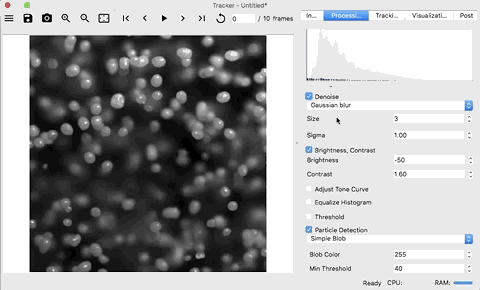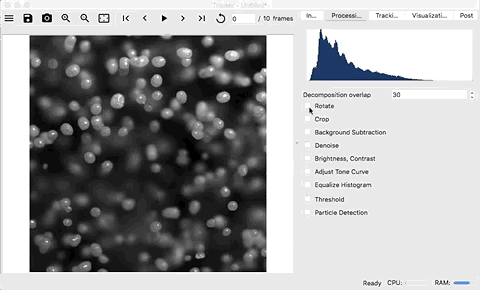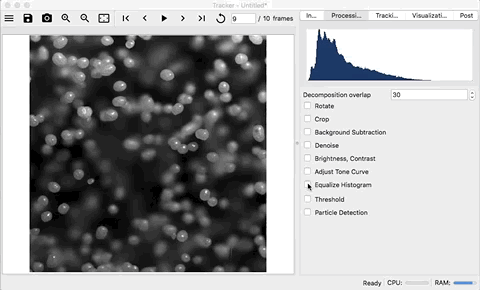# Process Images¶

After the media is opened, the individual frames are processed using a series of transforms. These transforms are applied and controlled on the Process tab. Unfortunately, which transforms to use depend heavily on the media. The basic goal is to maximize the contrast between the objects and the background. This process is very subjective, requiring trial and error.

Filters and transforms are applied sequentially in the order they are listed in. The results of individual steps can be seen by hovering over the filter or transform options.## Histogram¶

The first widget on the processing tab shows the histograms of the pre-processed frame (light gray) and the final processed image (dark blue) of the currently displayed frame. The histogram shows the normalized counts of pixels at a certain value. A counts of pixel values of 0 (black) are on the left side and counts of pixel values of 255 (white) are on the right side.

## Decomposition Overlap¶

When using parallel processing, there needs to be an overlap between neighboring tiles just larger then the largest object being tracked. Having an overlap too small will cause tracks to be lost while having an overlap to large will cause extra processing of pixels.

Note

To see the tiles, go the the visualization tab and select the Show domain decomposition check-box.

## Rotating¶

Frames can be rotated in increments of 90° by checking the Rotate check-box and selecting the amount of rotation to apply.## Cropping¶

Frames can be cropped by checking the Crop check-box and entering the amount of pixels to crop. The Left and Top entries are positive integers while the Right and Bottom entries are negative integers.## Background Subtraction¶

If there are structures in the image that do not move, these can be removed by using background subtraction. Background subtraction can be enabled by selecting the Background Subtraction check-box. There are three methods available:

1. mean - builds and updates an array of frames which is used to calculate a moving mean for each pixel. This mean is then subtracted from every frame. This method is implemented using numpy.

2. MOG2 - “Improved adaptive Gaussian mixture model for background subtraction” as implemented in OpenCV

3. KNN - “K-nearest neighbors - based Background/Foreground Segmentation” as implemented in OpenCV

See How to Use Background Subtraction Methods for more information.

Note

The history of the background subtraction methods is constructed as the frames are processed, so the first frame will typically be blank since there is no history.## Denoise¶

Noise can be removed from frames by selecting the Denoise check-box. There are three methods available:

1. Gaussian blur
2. Median blur
3. Bilateral filter

These three methods are implemented in OpenCV. See Smoothing Images for a tutorial with all three methods and more information.## Brightness and Contrast¶

The brightness and contrast of the frames can be adjusted using a linear transformation of the pixel values by selecting the Brightness, Contrast check-box:

$g(i,j)=\alpha⋅f(i,j)+\beta$

Where $$\alpha > 0$$ and $$\beta$$ are said to control contrast and brightness respectively. $$f(i,j)$$ is the pixel value of the input and $$g(i,j)$$ is the pixel value of the output.

See Changing the contrast and brightness of an image for more information.## Tone Curve¶

The brightness and contrast of the frames can be also be adjusted using a user controlled spline that is fit through 4 points, similar to tools found in Adobe’s Lightroom, by selecting the Adjust Tone curve check-box. This is similar to the linear transformation except that the input pixel values, $$f(i,j)$$, are transformed with a B-spline, $$h()$$, resulting in the pixel value of the output, $$g(i,j)$$:

$g(i,j)=h(f(i,j))$

Scipy’s splrep is used. This allows significant user control over increasing the contrast of the objects of interest.## Equalize Histogram¶

The contrast of the frames can be also be adjusted by using histogram equalization by selecting the Equalize Histogram check-box. Histogram equalization tries to stretch the intensity of the pixels to use the full range of available values (0-255). In some cases where the lighting is not uniform, adaptive histogram equalization can be used by selecting the Adaptive check-box. This will use the Contrast Limited Adaptive Histogram Equalization (CLAHE) technique as implemented in OpenCV. See Histograms - 2: Histogram Equalization for more information.## Threshold¶

A threshold can be applied to the frame by selecting the Threshold check-box. There are several types of thresholds that can be applied:

type condition
binary $$g(i,j)= 255 \text{ if } f(i,j)>thresh \text{ else } 0$$
binary inverted $$g(i,j)= 0 \text{ if } f(i,j)>thresh \text{ else } 255$$
truncated $$g(i,j)= thresh \text{ if } f(i,j)>thresh \text{ else } f(i,j)$$
to zero $$g(i,j)= f(i,j) \text{ if } f(i,j)>thresh \text{ else } 0$$
to zero inverted $$g(i,j)= 0 \text{ if } f(i,j)>thresh \text{ else } f(i,j)$$

Where $$f(i,j)$$ is the input pixel value, $$thresh$$ is the selected threshold value, and $$g(i,j)$$ is the resulting output pixel value.## Particle Detection¶

The last step in the processing of the images is the identification of the objects. To apply a object detection filter, select the Particle Detection check-box. There are three object detection filters including Simple Blob, Label, and Hough Circles.

### Simple Blob¶

Simple blob is an efficient algorithm for finding blobs in an image. It works by finding centers of contours at several thresholds from Min Threshold to Max Threshold, groups the centers, and then filters the blobs based on area, circularity, inertia, and/or convexity. This method is the preferred method for identifying objects.

### Label¶

If either a binary or binary inverted threshold is used, then the groups of pixels can be labeled . The centers of the labels can then be determined and used. This technique does not work well when objects are touching.

### Hough Circles¶

The Hough Circle Transform tries to find circles in an image. This transform works well if the objects are nice, fairly uniform circles, however it is sensitive to the input parameters and can be expensive. It can find overlapping circles.